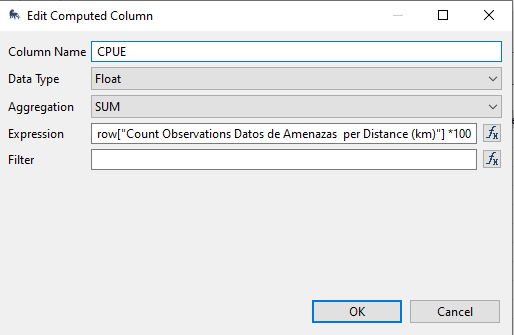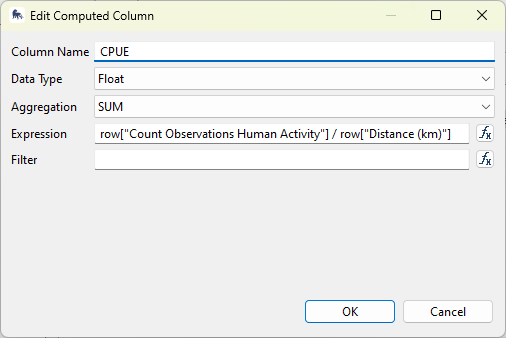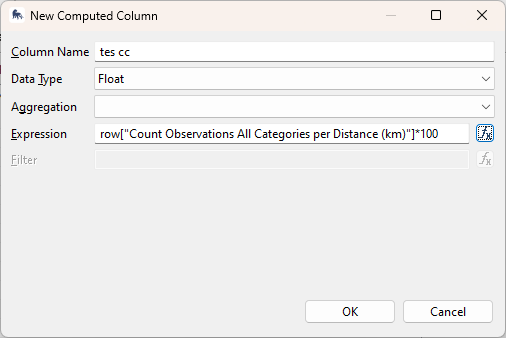# Computed column

Hello everyone:

I need help with this . I am generating this column that is the result of multiplying the frequency of observation of threats by distance patrolled to obtain the CPUE.
the formula is

when I run the report by year the result is correct

, but when i run for 2 years range de result of column CPUE is the sum of both year

This is the aggregationI think es very simple the solution, but i can´t find it.

Thanks

Hi Jorge,

As it’s generating the number it looks like the expression may be the issue. In this case I see that you’re using the existing CPUE generated through the query itself in the formula, which may be the problem.

You can try the formula as below. Although, the “compute rate” function in the query should remove the need to do this as a computed column in your report.

Hope this helps.

Cheers,

Alex1 Like

Hi Jval

You need to remove the SUM on aggregationRegards